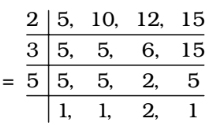Home » Aptitude » LCM and HCF » Question

#### LCM and HCF

1. The smallest number, which when divided by 5, 10, 12 and 15, leaves remainder 2 in each case; but when divided by 7 leaves no remainder, is
1. 189
2. 182
3. 175
4. 91
##### Correct Option: B

LCM of 5, 10, 12, 15∴ LCM = 2 × 3 × 5 × 2 = 60
∴ Number = 60k + 2
Now, the required number should be divisible by 7.
Now, 60k + 2 = 7 × 8k + 4k + 2
If we put k = 3 , (4k + 2) is equal to 14 which is exactly divisible by 7.
∴ Required number = 60 × 3 + 2 = 182